# 6.4 Graphs of logarithmic functions  (Page 8/8)

 Page 8 / 8

## Verbal

The inverse of every logarithmic function is an exponential function and vice-versa. What does this tell us about the relationship between the coordinates of the points on the graphs of each?

Since the functions are inverses, their graphs are mirror images about the line $\text{\hspace{0.17em}}y=x.\text{\hspace{0.17em}}$ So for every point $\text{\hspace{0.17em}}\left(a,b\right)\text{\hspace{0.17em}}$ on the graph of a logarithmic function, there is a corresponding point $\text{\hspace{0.17em}}\left(b,a\right)\text{\hspace{0.17em}}$ on the graph of its inverse exponential function.

What type(s) of translation(s), if any, affect the range of a logarithmic function?

What type(s) of translation(s), if any, affect the domain of a logarithmic function?

Shifting the function right or left and reflecting the function about the y-axis will affect its domain.

Consider the general logarithmic function $\text{\hspace{0.17em}}f\left(x\right)={\mathrm{log}}_{b}\left(x\right).\text{\hspace{0.17em}}$ Why can’t $\text{\hspace{0.17em}}x\text{\hspace{0.17em}}$ be zero?

Does the graph of a general logarithmic function have a horizontal asymptote? Explain.

No. A horizontal asymptote would suggest a limit on the range, and the range of any logarithmic function in general form is all real numbers.

## Algebraic

For the following exercises, state the domain and range of the function.

$f\left(x\right)={\mathrm{log}}_{3}\left(x+4\right)$

$h\left(x\right)=\mathrm{ln}\left(\frac{1}{2}-x\right)$

Domain: $\text{\hspace{0.17em}}\left(-\infty ,\frac{1}{2}\right);\text{\hspace{0.17em}}$ Range: $\text{\hspace{0.17em}}\left(-\infty ,\infty \right)$

$g\left(x\right)={\mathrm{log}}_{5}\left(2x+9\right)-2$

$h\left(x\right)=\mathrm{ln}\left(4x+17\right)-5$

Domain: $\text{\hspace{0.17em}}\left(-\frac{17}{4},\infty \right);\text{\hspace{0.17em}}$ Range: $\text{\hspace{0.17em}}\left(-\infty ,\infty \right)$

$f\left(x\right)={\mathrm{log}}_{2}\left(12-3x\right)-3$

For the following exercises, state the domain and the vertical asymptote of the function.

$\text{\hspace{0.17em}}f\left(x\right)={\mathrm{log}}_{b}\left(x-5\right)$

Domain: $\text{\hspace{0.17em}}\left(5,\infty \right);\text{\hspace{0.17em}}$ Vertical asymptote: $\text{\hspace{0.17em}}x=5$

$\text{\hspace{0.17em}}g\left(x\right)=\mathrm{ln}\left(3-x\right)$

$\text{\hspace{0.17em}}f\left(x\right)=\mathrm{log}\left(3x+1\right)$

Domain: $\text{\hspace{0.17em}}\left(-\frac{1}{3},\infty \right);\text{\hspace{0.17em}}$ Vertical asymptote: $\text{\hspace{0.17em}}x=-\frac{1}{3}$

$\text{\hspace{0.17em}}f\left(x\right)=3\mathrm{log}\left(-x\right)+2$

$\text{\hspace{0.17em}}g\left(x\right)=-\mathrm{ln}\left(3x+9\right)-7$

Domain: $\text{\hspace{0.17em}}\left(-3,\infty \right);\text{\hspace{0.17em}}$ Vertical asymptote: $\text{\hspace{0.17em}}x=-3$

For the following exercises, state the domain, vertical asymptote, and end behavior of the function.

$f\left(x\right)=\mathrm{ln}\left(2-x\right)$

$f\left(x\right)=\mathrm{log}\left(x-\frac{3}{7}\right)$

Domain: $\left(\frac{3}{7},\infty \right)$ ;
Vertical asymptote: $x=\frac{3}{7}$ ; End behavior: as $x\to {\left(\frac{3}{7}\right)}^{+},f\left(x\right)\to -\infty$ and as $x\to \infty ,f\left(x\right)\to \infty$

$h\left(x\right)=-\mathrm{log}\left(3x-4\right)+3$

$g\left(x\right)=\mathrm{ln}\left(2x+6\right)-5$

Domain: $\left(-3,\infty \right)$ ; Vertical asymptote: $x=-3$ ;
End behavior: as $x\to -{3}^{+}$ , $f\left(x\right)\to -\infty$ and as $x\to \infty$ , $f\left(x\right)\to \infty$

$f\left(x\right)={\mathrm{log}}_{3}\left(15-5x\right)+6$

For the following exercises, state the domain, range, and x - and y -intercepts, if they exist. If they do not exist, write DNE.

$h\left(x\right)={\mathrm{log}}_{4}\left(x-1\right)+1$

Domain: $\text{\hspace{0.17em}}\left(1,\infty \right);\text{\hspace{0.17em}}$ Range: $\text{\hspace{0.17em}}\left(-\infty ,\infty \right);\text{\hspace{0.17em}}$ Vertical asymptote: $\text{\hspace{0.17em}}x=1;\text{\hspace{0.17em}}$ x -intercept: $\text{\hspace{0.17em}}\left(\frac{5}{4},0\right);\text{\hspace{0.17em}}$ y -intercept: DNE

$f\left(x\right)=\mathrm{log}\left(5x+10\right)+3$

$g\left(x\right)=\mathrm{ln}\left(-x\right)-2$

Domain: $\text{\hspace{0.17em}}\left(-\infty ,0\right);\text{\hspace{0.17em}}$ Range: $\text{\hspace{0.17em}}\left(-\infty ,\infty \right);\text{\hspace{0.17em}}$ Vertical asymptote: $\text{\hspace{0.17em}}x=0;\text{\hspace{0.17em}}$ x -intercept: $\text{\hspace{0.17em}}\left(-{e}^{2},0\right);\text{\hspace{0.17em}}$ y -intercept: DNE

$f\left(x\right)={\mathrm{log}}_{2}\left(x+2\right)-5$

$h\left(x\right)=3\mathrm{ln}\left(x\right)-9$

Domain: $\text{\hspace{0.17em}}\left(0,\infty \right);\text{\hspace{0.17em}}$ Range: $\text{\hspace{0.17em}}\left(-\infty ,\infty \right);\text{\hspace{0.17em}}$ Vertical asymptote: $\text{\hspace{0.17em}}x=0;\text{\hspace{0.17em}}$ x -intercept: $\text{\hspace{0.17em}}\left({e}^{3},0\right);\text{\hspace{0.17em}}$ y -intercept: DNE

## Graphical

For the following exercises, match each function in [link] with the letter corresponding to its graph.

$d\left(x\right)=\mathrm{log}\left(x\right)$

$f\left(x\right)=\mathrm{ln}\left(x\right)$

B

$g\left(x\right)={\mathrm{log}}_{2}\left(x\right)$

$h\left(x\right)={\mathrm{log}}_{5}\left(x\right)$

C

$j\left(x\right)={\mathrm{log}}_{25}\left(x\right)$

For the following exercises, match each function in [link] with the letter corresponding to its graph.

$f\left(x\right)={\mathrm{log}}_{\frac{1}{3}}\left(x\right)$

B

$g\left(x\right)={\mathrm{log}}_{2}\left(x\right)$

$h\left(x\right)={\mathrm{log}}_{\frac{3}{4}}\left(x\right)$

C

For the following exercises, sketch the graphs of each pair of functions on the same axis.

$f\left(x\right)=\mathrm{log}\left(x\right)\text{\hspace{0.17em}}$ and $\text{\hspace{0.17em}}g\left(x\right)={10}^{x}$

$f\left(x\right)=\mathrm{log}\left(x\right)\text{\hspace{0.17em}}$ and $\text{\hspace{0.17em}}g\left(x\right)={\mathrm{log}}_{\frac{1}{2}}\left(x\right)$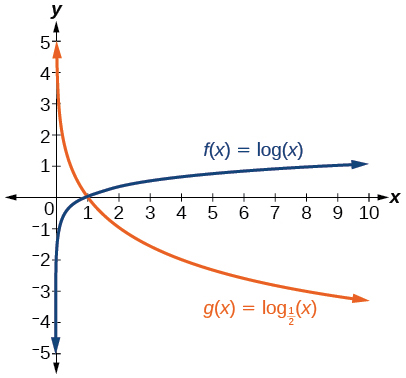$f\left(x\right)={\mathrm{log}}_{4}\left(x\right)\text{\hspace{0.17em}}$ and $\text{\hspace{0.17em}}g\left(x\right)=\mathrm{ln}\left(x\right)$

$f\left(x\right)={e}^{x}\text{\hspace{0.17em}}$ and $\text{\hspace{0.17em}}g\left(x\right)=\mathrm{ln}\left(x\right)$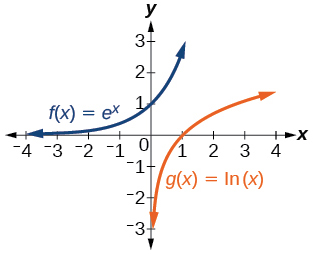For the following exercises, match each function in [link] with the letter corresponding to its graph.

$f\left(x\right)={\mathrm{log}}_{4}\left(-x+2\right)$

$g\left(x\right)=-{\mathrm{log}}_{4}\left(x+2\right)$

C

$h\left(x\right)={\mathrm{log}}_{4}\left(x+2\right)$

For the following exercises, sketch the graph of the indicated function.

$\text{\hspace{0.17em}}f\left(x\right)={\mathrm{log}}_{2}\left(x+2\right)$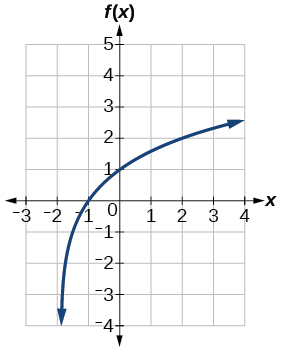$\text{\hspace{0.17em}}f\left(x\right)=2\mathrm{log}\left(x\right)$

$\text{\hspace{0.17em}}f\left(x\right)=\mathrm{ln}\left(-x\right)$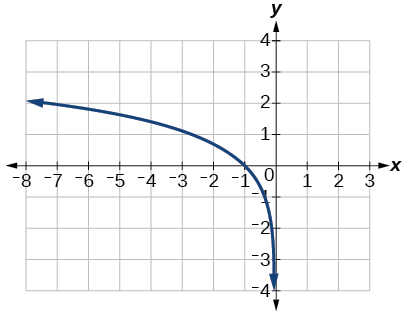$g\left(x\right)=\mathrm{log}\left(4x+16\right)+4$

$g\left(x\right)=\mathrm{log}\left(6-3x\right)+1$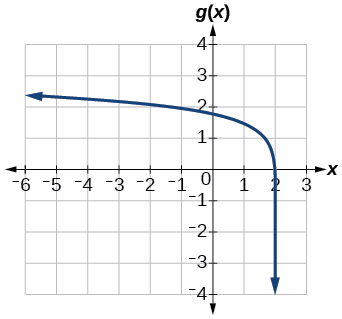$h\left(x\right)=-\frac{1}{2}\mathrm{ln}\left(x+1\right)-3$

For the following exercises, write a logarithmic equation corresponding to the graph shown.

Use $\text{\hspace{0.17em}}y={\mathrm{log}}_{2}\left(x\right)\text{\hspace{0.17em}}$ as the parent function.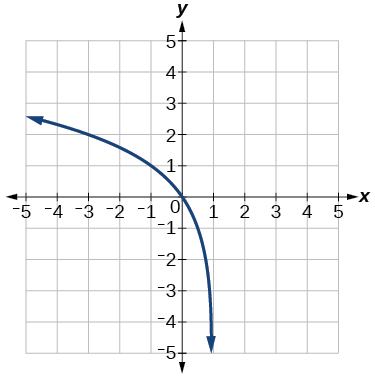$\text{\hspace{0.17em}}f\left(x\right)={\mathrm{log}}_{2}\left(-\left(x-1\right)\right)$

Use $\text{\hspace{0.17em}}f\left(x\right)={\mathrm{log}}_{3}\left(x\right)\text{\hspace{0.17em}}$ as the parent function.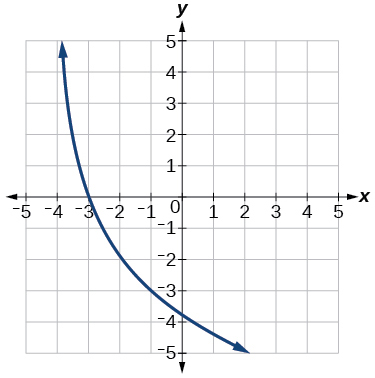Use $\text{\hspace{0.17em}}f\left(x\right)={\mathrm{log}}_{4}\left(x\right)\text{\hspace{0.17em}}$ as the parent function.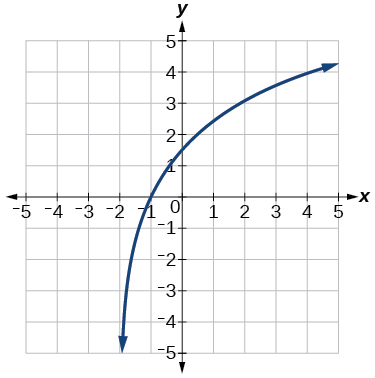$f\left(x\right)=3{\mathrm{log}}_{4}\left(x+2\right)$

Use $\text{\hspace{0.17em}}f\left(x\right)={\mathrm{log}}_{5}\left(x\right)\text{\hspace{0.17em}}$ as the parent function.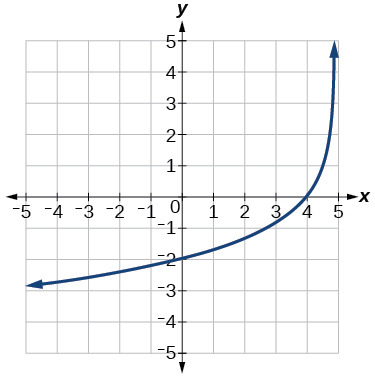## Technology

For the following exercises, use a graphing calculator to find approximate solutions to each equation.

$\mathrm{log}\left(x-1\right)+2=\mathrm{ln}\left(x-1\right)+2$

$x=2$

$\mathrm{log}\left(2x-3\right)+2=-\mathrm{log}\left(2x-3\right)+5$

$\mathrm{ln}\left(x-2\right)=-\mathrm{ln}\left(x+1\right)$

$x\approx \text{2}\text{.303}$

$2\mathrm{ln}\left(5x+1\right)=\frac{1}{2}\mathrm{ln}\left(-5x\right)+1$

$\frac{1}{3}\mathrm{log}\left(1-x\right)=\mathrm{log}\left(x+1\right)+\frac{1}{3}$

$x\approx -0.472$

## Extensions

Let $\text{\hspace{0.17em}}b\text{\hspace{0.17em}}$ be any positive real number such that $\text{\hspace{0.17em}}b\ne 1.\text{\hspace{0.17em}}$ What must $\text{\hspace{0.17em}}{\mathrm{log}}_{b}1\text{\hspace{0.17em}}$ be equal to? Verify the result.

Explore and discuss the graphs of $\text{\hspace{0.17em}}f\left(x\right)={\mathrm{log}}_{\frac{1}{2}}\left(x\right)\text{\hspace{0.17em}}$ and $\text{\hspace{0.17em}}g\left(x\right)=-{\mathrm{log}}_{2}\left(x\right).\text{\hspace{0.17em}}$ Make a conjecture based on the result.

The graphs of $\text{\hspace{0.17em}}f\left(x\right)={\mathrm{log}}_{\frac{1}{2}}\left(x\right)\text{\hspace{0.17em}}$ and $\text{\hspace{0.17em}}g\left(x\right)=-{\mathrm{log}}_{2}\left(x\right)\text{\hspace{0.17em}}$ appear to be the same; Conjecture: for any positive base $\text{\hspace{0.17em}}b\ne 1,$ $\text{\hspace{0.17em}}{\mathrm{log}}_{b}\left(x\right)=-{\mathrm{log}}_{\frac{1}{b}}\left(x\right).$

Prove the conjecture made in the previous exercise.

What is the domain of the function $\text{\hspace{0.17em}}f\left(x\right)=\mathrm{ln}\left(\frac{x+2}{x-4}\right)?\text{\hspace{0.17em}}$ Discuss the result.

Recall that the argument of a logarithmic function must be positive, so we determine where $\text{\hspace{0.17em}}\frac{x+2}{x-4}>0\text{\hspace{0.17em}}$ . From the graph of the function $\text{\hspace{0.17em}}f\left(x\right)=\frac{x+2}{x-4},$ note that the graph lies above the x -axis on the interval $\text{\hspace{0.17em}}\left(-\infty ,-2\right)\text{\hspace{0.17em}}$ and again to the right of the vertical asymptote, that is $\text{\hspace{0.17em}}\left(4,\infty \right).\text{\hspace{0.17em}}$ Therefore, the domain is $\text{\hspace{0.17em}}\left(-\infty ,-2\right)\cup \left(4,\infty \right).$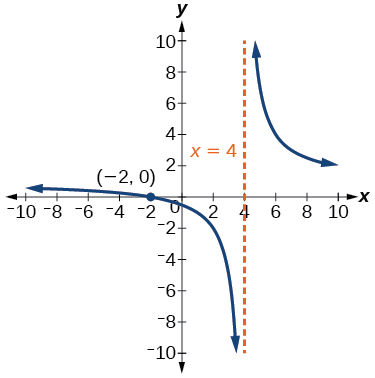Use properties of exponents to find the x -intercepts of the function $\text{\hspace{0.17em}}f\left(x\right)=\mathrm{log}\left({x}^{2}+4x+4\right)\text{\hspace{0.17em}}$ algebraically. Show the steps for solving, and then verify the result by graphing the function.

By the definition, is such that 0!=1.why?
(1+cosA+IsinA)(1+cosB+isinB)/(cos@+isin@)(cos$+isin$)
hatdog
Mark
how we can draw three triangles of distinctly different shapes. All the angles will be cutt off each triangle and placed side by side with vertices touching
bsc F. y algebra and trigonometry pepper 2
given that x= 3/5 find sin 3x
4
DB
remove any signs and collect terms of -2(8a-3b-c)
-16a+6b+2c
Will
Joeval
(x2-2x+8)-4(x2-3x+5)
sorry
Miranda
x²-2x+9-4x²+12x-20 -3x²+10x+11
Miranda
x²-2x+9-4x²+12x-20 -3x²+10x+11
Miranda
(X2-2X+8)-4(X2-3X+5)=0 ?
master
The anwser is imaginary number if you want to know The anwser of the expression you must arrange The expression and use quadratic formula To find the answer
master
The anwser is imaginary number if you want to know The anwser of the expression you must arrange The expression and use quadratic formula To find the answer
master
Y
master
master
Soo sorry (5±Root11* i)/3
master
Mukhtar
2x²-6x+1=0
Ife
explain and give four example of hyperbolic function
What is the correct rational algebraic expression of the given "a fraction whose denominator is 10 more than the numerator y?
y/y+10
Mr
Find nth derivative of eax sin (bx + c).
Find area common to the parabola y2 = 4ax and x2 = 4ay.
Anurag
y2=4ax= y=4ax/2. y=2ax
akash
A rectangular garden is 25ft wide. if its area is 1125ft, what is the length of the garden
to find the length I divide the area by the wide wich means 1125ft/25ft=45
Miranda
thanks
Jhovie
What do you call a relation where each element in the domain is related to only one value in the range by some rules?
A banana.
Yaona
a function
Daniel
a function
emmanuel
given 4cot thither +3=0and 0°<thither <180° use a sketch to determine the value of the following a)cos thither
what are you up to?
nothing up todat yet
Miranda
hi
jai
hello
jai
Miranda Drice
jai
aap konsi country se ho
jai
which language is that
Miranda
I am living in india
jai
good
Miranda
what is the formula for calculating algebraic
I think the formula for calculating algebraic is the statement of the equality of two expression stimulate by a set of addition, multiplication, soustraction, division, raising to a power and extraction of Root. U believe by having those in the equation you will be in measure to calculate it
Miranda

#### Get Jobilize Job Search Mobile App in your pocket Now!ByByBy Angelica LitoBy Saylor FoundationBy Steve GibbsBy Richley CrapoBy OpenStaxBy Anh DaoBy Janet ForresterBy Jonathan LongBy Anh DaoBy OpenStax